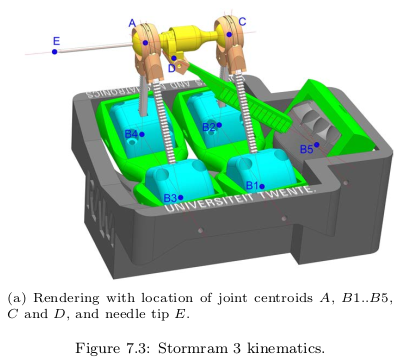## Stormram

One of my friends (Vincent Groenhuis) has obtained a PhD for research on medical robots that can position a biopsy needle.
In one of the models, called "Stormram 1", the position of the needle ($$\vec E$$) depends on the positions of 5 pistons, stemming from points $$\vec B_1$$ till $$\vec B_5$$.
I took up the challenge to work this calculation out, and test its convergence. That could save the purchase of a Matlab licence!

(click on the picture below to rotate it)

 R1 (from B1 to C) R2 (from B2 to C)
 R3 (from B3 to A) R4 (from B4 to A)
 R5 (from B5 to D)(This picture was taken from Robotic systems for breast biopsy using MRI and ultrasound imaging ISBN: 978-90-365-4892-2 by Vincent Groenhuis. I thank the author for his permission)
The calculation took some programming. If we know the distance from $$B_5$$ to $$A$$, we can calculate the position of $$\vec A$$.
To know this distance, we must know the position of $$\vec D$$. But $$\vec D$$ can rotate around the axis $$\vec A-\vec C$$, so it depends on the position of $$\vec A$$.

This sounds like a circle without end!
The solution is explained here.